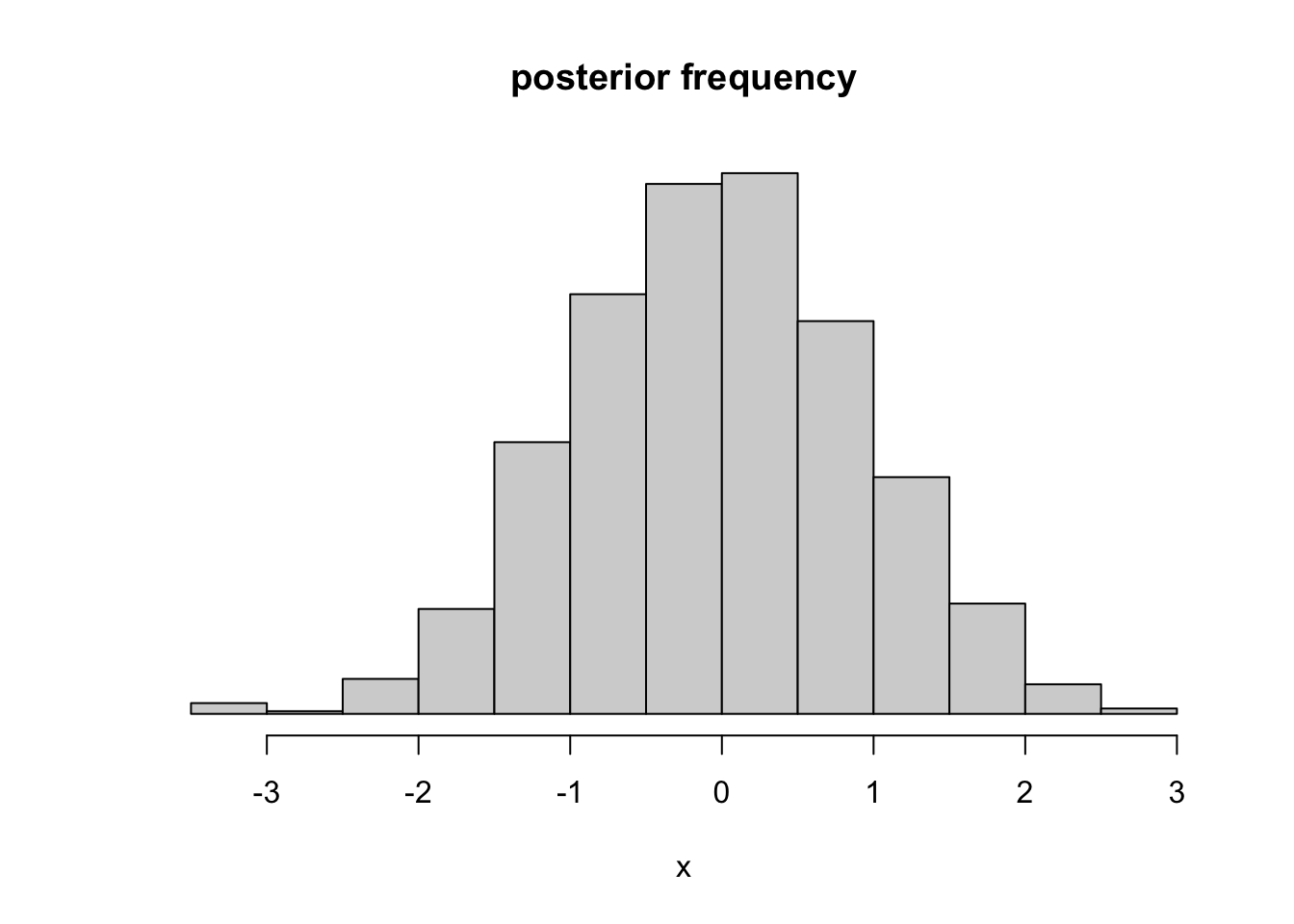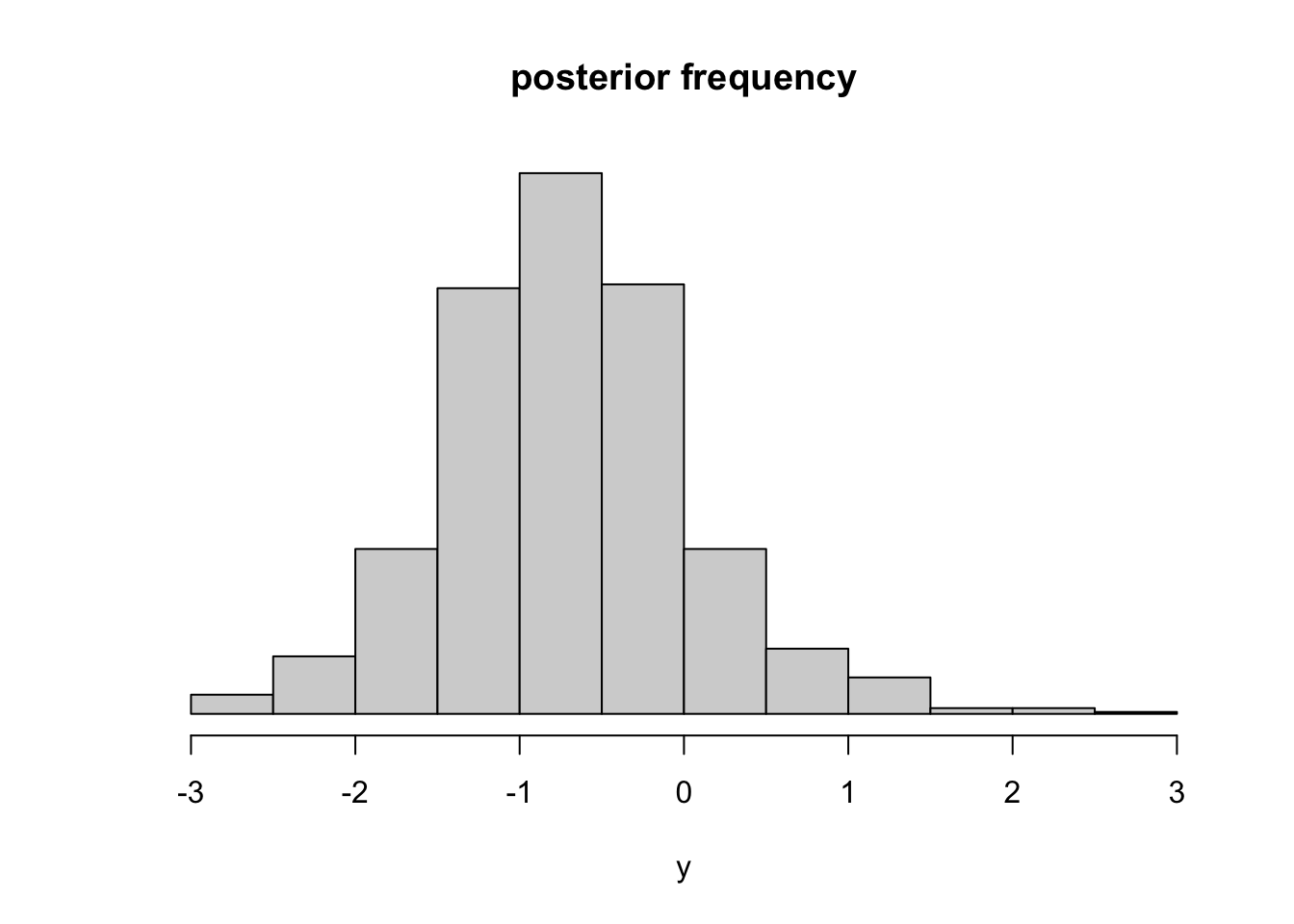Jacobian adjustments are a persistent source of confusion for applied Bayesian modelers. What do they do? When are they necessary? Most explanations floating around the internet assume familiarity with the idea that nonlinear transformations cause “distortion” that requires an “adjustment for the curvature.” If this is you, read no further. Instead, ponder the implications of integration by substitution for probability density functions. Or for a treatment that explicitly links probability distributions, probability density functions, and Jacobian adjustments, check out Michael Betancourt on probability theory (highly recommended!). For Stan-specific treatments, check out the Users Guide or Kazuki Yoshida’s explanation.

If these treatments leave you confused, don’t despair! We can build strong intuition about Jacobian adjustments by setting aside most of the math and just reasoning about the probability densities in your model. The math won’t get any worse than taking a derivative. Best of all, you’ll gain a deeper understanding of what it means to “parametrize” a model, and the role of Stan’s parameters block.

### A motivating example

Take the following Stan program, a model with no data that places a normal prior on $$x$$.

parameters{
real x;
}
model{
x ~ std_normal();
}

Sampling from this model yields a standard normal as the posterior distribution for $$x$$.But now consider this next model, which appears to place a standard normal prior on $$e^x + \frac{x}{10}$$. This seems like an obscure choice for a tranform, but I promise I have my reasons1.

parameters{
real x;
}
transformed parameters{
real y = exp(x) + x/10;
}
model{
y ~ std_normal();
}

We get divergent transitions when we fit this thing, indicating that we’ve done something nasty to the posterior geometry, but we can eliminate the divergences and get a trustworthy posterior by increasing adapt_delta to $$0.999$$. When we do, we see that the posterior for $$y = e^x + \frac{x}{10}$$ is not a standard normal!This post is the story of where that normal distribution went and how we can get it back.

### The target density

For a given parametrization, a posterior distribution is encoded by a probability density function (PDF) over the parameters2. The goal of MCMC sampling is to draw samples from this posterior PDF. The purpose of a Stan program is to specify a density function that is proportional to the posterior PDF. Stan calls this density the target density.

In a Stan program, “sampling statements” like x ~ std_normal() serve to modify the target density (in this case multiplying it by the PDF3 associated with the standard normal distribution).

An important aside here is that Stan doesn’t work directly with the target density, but rather with its logarithm. Thus, rather than multiplying the target density by a PDF, what Stan’s sampling statements really do is to add the logarithm of a PDF to the logarithm of the target density. With that in mind, it’s time to introduce a really cool piece of Stan syntax: target +=. In Stan, the logarithm of the target density is stored in a variable called target, and += means to take the variable on the left and increment it by the variable on the right.4 Thus, instead of writing x ~ std_normal(), we could instead choose to write target += normal_lpdf(x | 0, 1), which in English means “increment the logarithm of the target density by the logarithm of the PDF (as a function of $$x$$) that encodes $$Normal(0,1)$$”.

Since the target density just needs to be proportional to the posterior, it doesn’t matter where the target density “starts” (before it gets incremented). Stan initializes the target density at 1 (i.e. it initializes the log density at 0), but it could just as well start at any positive value as long as the initial density is flat.5

### Transforming uniform densities

When we apply a nonlinear transform to a parameter with a uniform density (i.e. a flat density), the density of the transform is not uniform. Let’s take a look at $$y=f(x)=e^x + \frac{x}{10}$$. Look at how equal intervals of $$x$$ transform to unequal intervals of $$y$$.